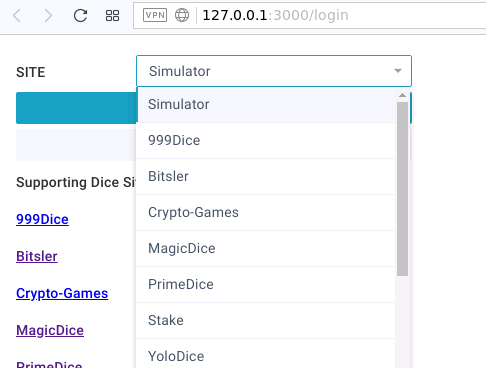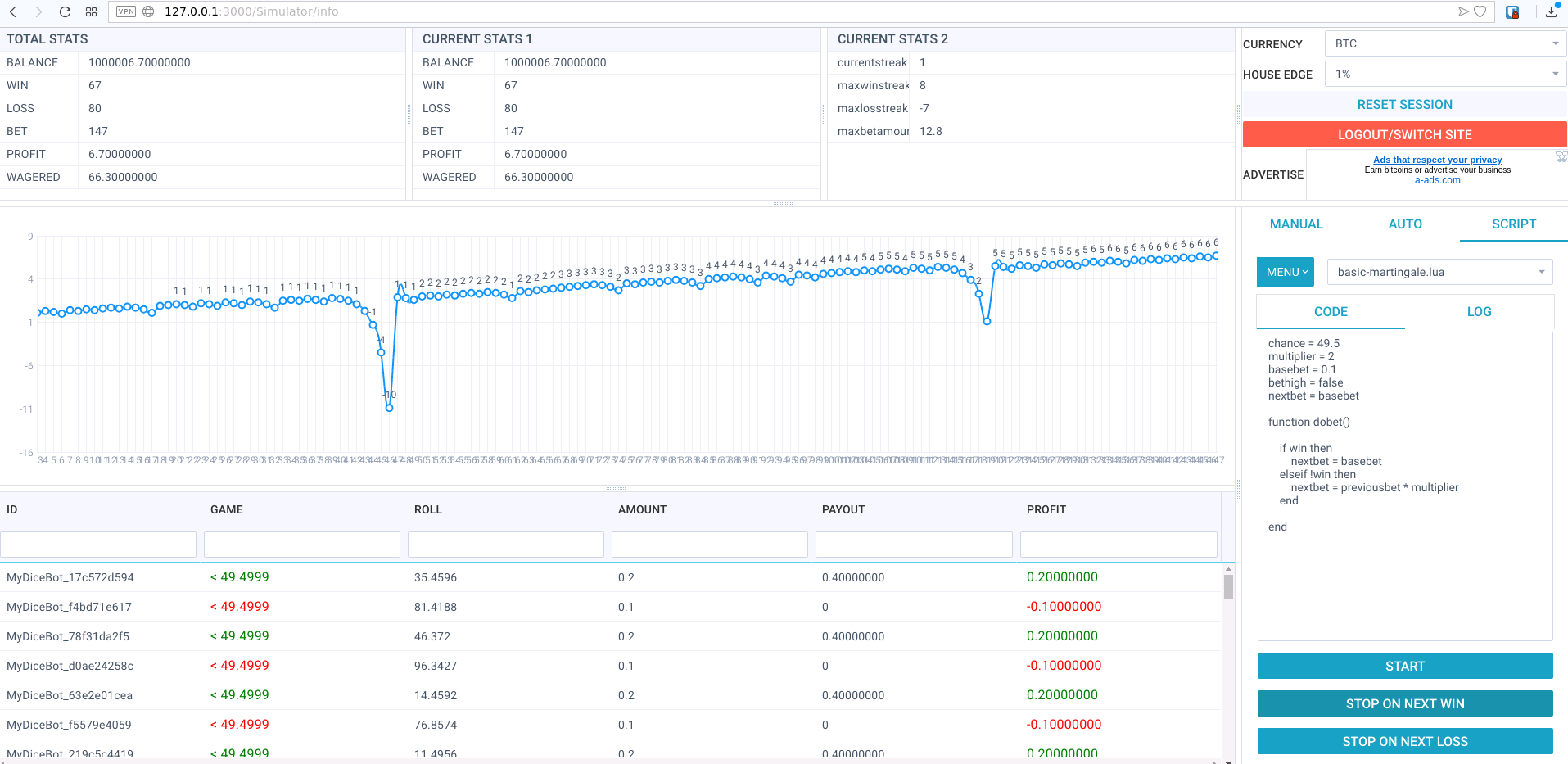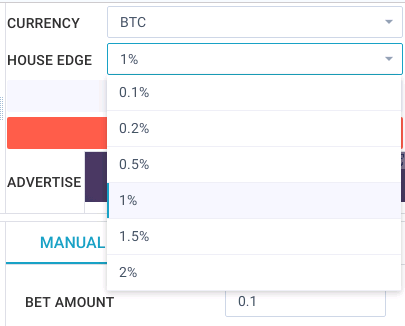# MyDiceBot - 190122 is released & Simulator is available NOW!# MyDiceBot

• https://mydicebot.com
• MyDiceBot is World #1 Cross-Platform Dicing Bot.
• Multiple platforms are supported, including Windows, Mac, Linux, and Web.
• Multiple blockchains are supported, including STEEM.
• Multiple programming languages are supported such as Lua.
• Open Source and Free Forever

# Major Feature update

• Simulator is available NOW!• You could debugging your own script in Simulator without any risk.• You can adjust house edge to debug against.• Server Simulation Code
``````  async _simulatorBet(amount, target, condition, houseEdge ) {
let betInfo = {};
betInfo.id = 'MyDiceBot_'+Math.random().toString(16).substring(2).substr(0,10);
betInfo.amount = parseFloat(amount);
let serverSeed = Math.random().toString(36).substring(2);
let clientSeed = Math.random().toString(36).substring(2);
let cryptoHmac = crypto.createHmac('sha256', serverSeed);
let resultSeed = cryptoHmac.update(`\${clientSeed}`).digest('hex');
let resultNumber = parseInt(resultSeed.substr(0, 10), 16);
let diceRoll = (resultNumber % 1000000)+1;
let factor = 1000000/(target+1);
if(condition == 'over') {
factor = 1000000/(999999-target+1);
}
let profit = (amount * factor) * (1 - houseEdge) - amount;
console.log(amount,factor,betInfo.amount, houseEdge);
betInfo.roll_number = diceRoll/10000;
betInfo.win = false;
if(condition == 'over') {
if(target<diceRoll) {
betInfo.win = true;
}
} else {
if(target>diceRoll){
betInfo.win = true;
}
}
if(betInfo.win) {
betInfo.payout = parseFloat((betInfo.amount+profit)).toFixed(8);
betInfo.profit = parseFloat(profit).toFixed(8);
} else {
betInfo.payout = 0;
betInfo.profit = parseFloat(-betInfo.amount).toFixed(8);
}
console.log(betInfo);
return betInfo;
}
``````

# Improvement - Default embedded scripts for easy usage

• basic-martingale.lua ```lua chance = 49.5 multiplier = 2 basebet = 0.00000001 bethigh = false nextbet = basebet

function dobet()

``````if win then
nextbet = basebet
elseif !win then
nextbet = previousbet * multiplier
end
``````

end

``````* chance-10.lua
```lua
chance = 10
nextbet = 0.00001000
basebet = 0.00001000
installBet = 0.00001000
X = 1.101
memberbet = 0
breakpoint = 0
bethigh = true
game = true
regame = true

function dobet()
if balance >= breakpoint then
breakpoint = balance
end

if bethigh and currentroll < chance then
X = X + 0.001
end

if !bethigh and currentroll > (100-chance) then
X = X + 0.001
end

if game then
if !win then
installBet = previousbet*X
end
if win then
installBet = memberbet
end
if currentstreak < -10 then
installBet = 0.00001000
game = false
regame = false
end
nextbet = installBet
end
if !game and currentstreak < -20 then
regame = true
end
if win and regame then
game = true
nextbet = memberbet
end
if win and balance >= breakpoint then
memberbet = 0.00001000
X = 1.101
game = true
nextbet = 0.00001000
end
if previousbet >= memberbet then
memberbet = previousbet
end
print(breakpoint)
print('mult '..X)
print('memberbet '..memberbet)
end
``````
• regression.lua ```lua –[[ This script doubles the bet after a loss but starts at a very high chance of success: 95%.
After each bet the chance reduces enough to bring equilibrium to profit but stops reducing chance at 49.5%.
First loss goes from 95% to 67%, then 57% and then gradually lower and closer to 49.5%.
It basically buys you a few more higher probability roles before the same old 49.5% martingale. author: grendel25aaaaa link: https://bot.seuntjie.com/scripts.aspx?id=38 ]]

– You could just use Sleep(n) to use LUAs built in sleep function. – But this one will have the same result without killing your CPU. function sleep(n) t0 = os.clock() while os.clock() - t0 <= n do end end

– init chance = 95 basebet = 0.00000100 bethigh = false lossstreakcount = 0 – sample: user-defined lossstreakcount. nextbet = basebet

– do bet and let’s rock function dobet()

``````-- some sites limit bet latency due to the low bet amount.
-- enable it and avoid to be banned, just in case.
-- eg. 2 means sleeping 2 seconds
-- sleep(2)

-- adjust the stopping condition of session profit.
if profit >= 0.00001000 then
print("profit done, so stop.")
stop()
end

-- adjust the stopping condition of wins.
if wins >= 1000 then
print("wins done, so stop.")
stop()
end

-- adjust the stopping condition of bets.
if (bets >= 5000) then
print("bets done, so stop.")
stop()
end

-- adjust the stopping condition of loss streak.
-- eg. -10 means 10 loss streak
if (currentstreak <= -10) then
print("10 loss streak, so stop.")
stop()
end

-- if win, reset bet to base amount.
if (win) then
chance = 95
nextbet = basebet
lossstreakcount = 0
print("WIN")
end

-- if loss,
-- first loss goes from 95% to 67%,
-- then 57% and then gradually lower and closer to 49.5%.
if (!win) then
lossstreakcount += 1
if (lossstreakcount > 1) then
nextbet = previousbet*2
chance = (1/(((nextbet+(nextbet-basebet))/nextbet)))*100
if chance < 49.5 then chance = 49.5 end
bethigh = !bethigh
print ("LOSE")
else
nextbet = previousbet*2
chance = (1/(((basebet+nextbet))/nextbet))*100
if chance < 49.5 then chance = 49.5 end
bethigh = !bethigh
print ("LOSE")
end
end end ```
``````

# Quick Start

• Download MyDiceBot Binaries here: MyDiceBot Releases.
• Different execution methods on different platforms.
• Linux (Open Terminal)

``````  chmod +x mydicebot-linux
``````
``````  ./mydicebot-linux
``````
• Mac (Open Terminal)

``````  chmod +x mydicebot-macos
``````
``````  ./mydicebot-macos
``````
• Windows (Open Command Prompt)

``````  mydicebot-win.exe
``````